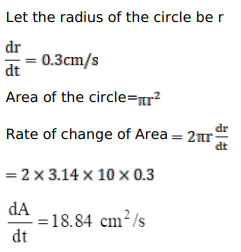# The radius of a circle is increasing uniformly at the rate of

Question:

The radius of a circle is increasing uniformly at the rate of $0.3$ centimetre per second. At what rate is the area increasing when the radius is $10 \mathrm{~cm}$ ?

(Take $\pi=3.14 .)$

Solution: